# Line graph with multiple lines in ggplot2

## Data transformation

Consider the following data frame where each column represents the path of a brownian motion.

``````# Brownian motion
set.seed(2)

# Grid
t <- seq(0, 1, by = 0.001)
p <- length(t) - 1

# 5 paths
n <- 5
I <- matrix(rnorm(n * p, 0, 1 / sqrt(p)), n, p)

# Data frame
df1 <- data.frame(apply(I, 1, cumsum))``````

In order to use your data frame in ggplot2 you will need to transform it into long format. You can achieve it making use of the `melt` function of the `reshape` package.

``````# install.packages("reshape")
library(reshape)

df <- data.frame(x = seq_along(df1[, 1]),
df1)

# Long format
df <- melt(df, id.vars = "x")``````

## Line chart of several variables

Given a data frame in long format like `df` it is possible to create a line chart with multiple lines in ggplot2 with `geom_line` the following way.

``````# install.packages("ggplot2")
library(ggplot2)

ggplot(df, aes(x = x, y = value, color = variable)) +
geom_line()``````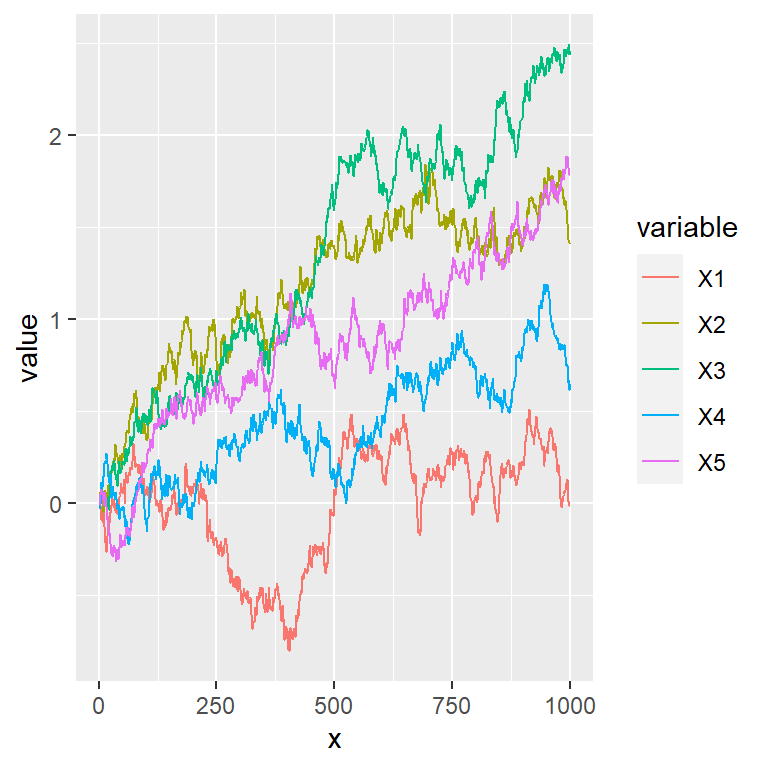Lines width and style

The styling of the lines can be changed making use of the arguments of `geom_line`, like `linetype` for changing the style of the line or `lwd` to change its width.

``````# install.packages("ggplot2")
library(ggplot2)

ggplot(df, aes(x = x, y = value, color = variable)) +
geom_line(linetype = 3,
lwd = 1.1)``````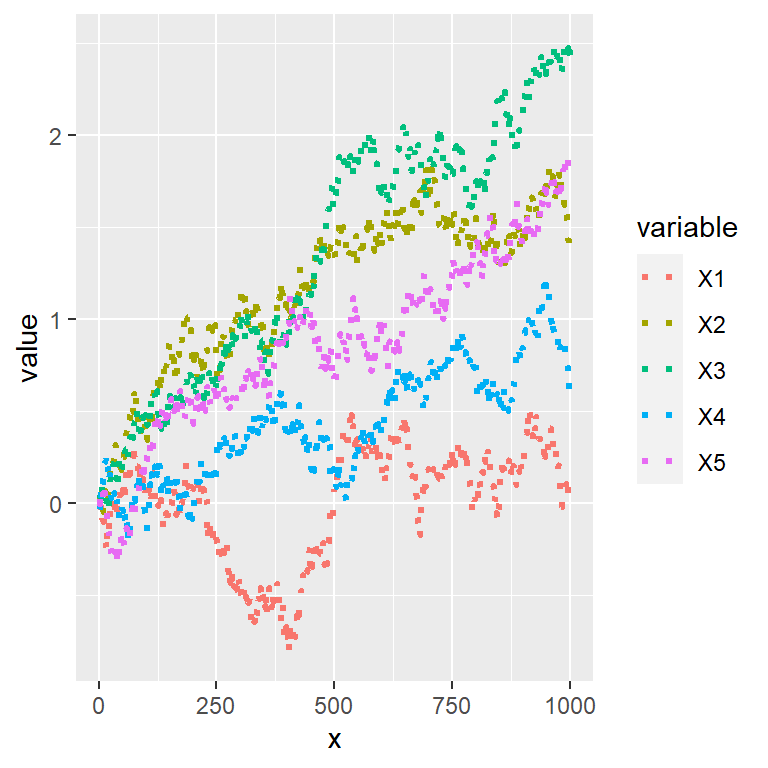Color customization

The default color palette can be changed passing a vector of colors to the `values` argument of the `scale_color_manual` function.

``````# install.packages("ggplot2")
library(ggplot2)

# Color selection
cols <- c("#D43F3A", "#EEA236", "#5CB85C", "#46B8DA", "#9632B8")

ggplot(df, aes(x = x, y = value, color = variable)) +
geom_line() +
scale_color_manual(values = cols)``````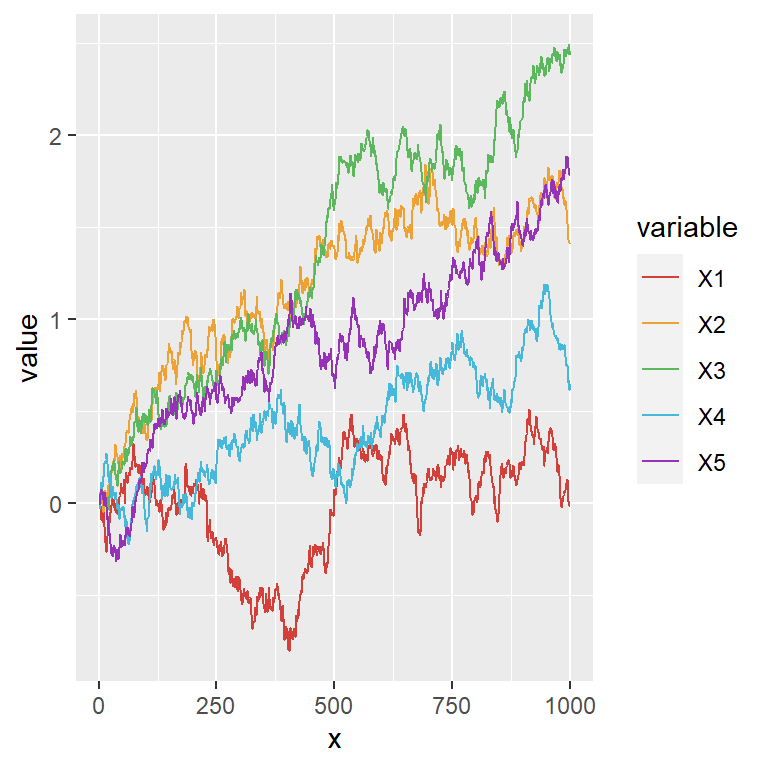Highlight some lines

Note that using the previous method you can also highlight some lines of the chart, using the same color for all lines but some.

``````# install.packages("ggplot2")
library(ggplot2)

# Color selection
cols <- c("gray", "gray", "gray", "#5CB85C", "gray")

ggplot(df, aes(x = x, y = value, color = variable)) +
geom_line() +
scale_color_manual(values = cols)``````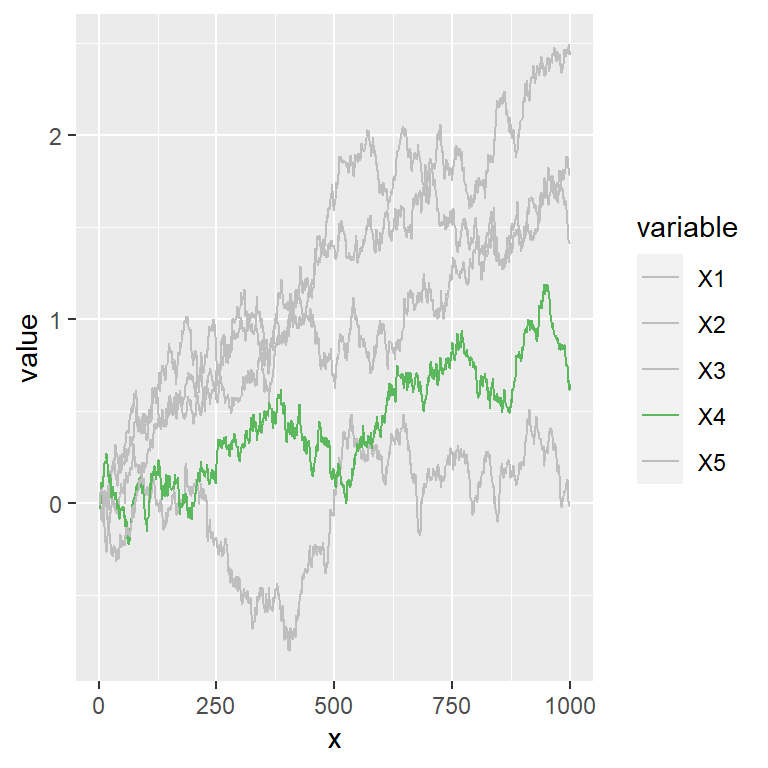## Legend customization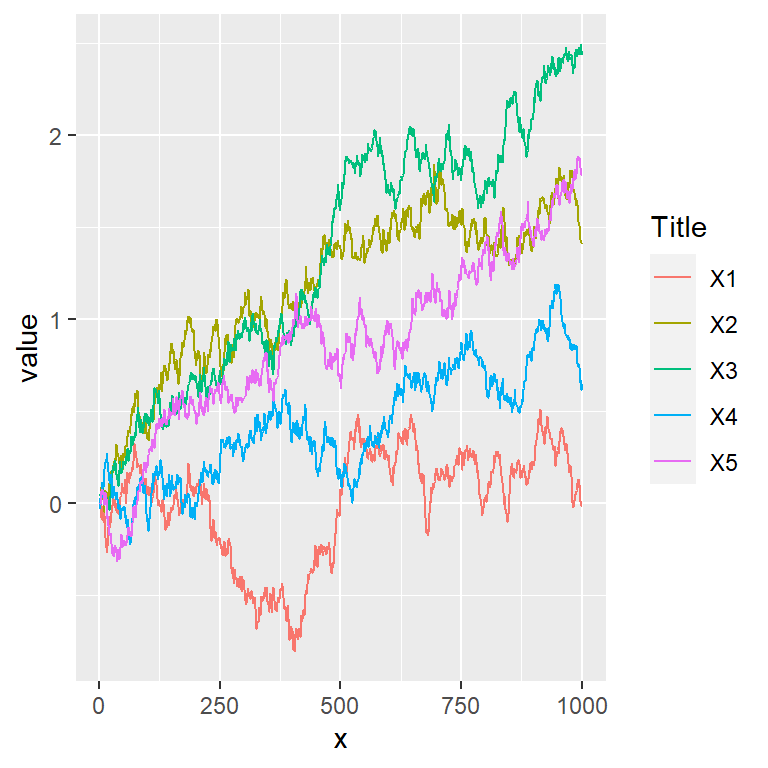Title

You can change the default title for the legend of the line chart with `guide_legend` as shown below.

``````# install.packages("ggplot2")
library(ggplot2)

ggplot(df, aes(x = x, y = value, color = variable)) +
geom_line() +
guides(color = guide_legend(title = "Title"))``````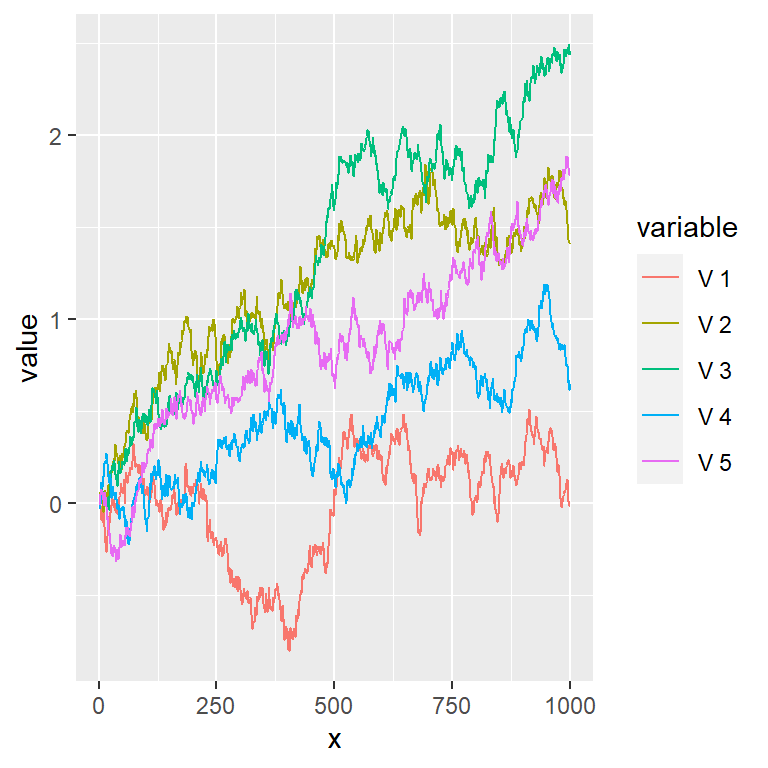Legend labels

The labels of the legend can be modified making use of the `labels` argument of `scale_color_discrete`.

``````# install.packages("ggplot2")
library(ggplot2)

ggplot(df, aes(x = x, y = value, color = variable)) +
geom_line() +
scale_color_discrete(labels = paste("V", 1:5))``````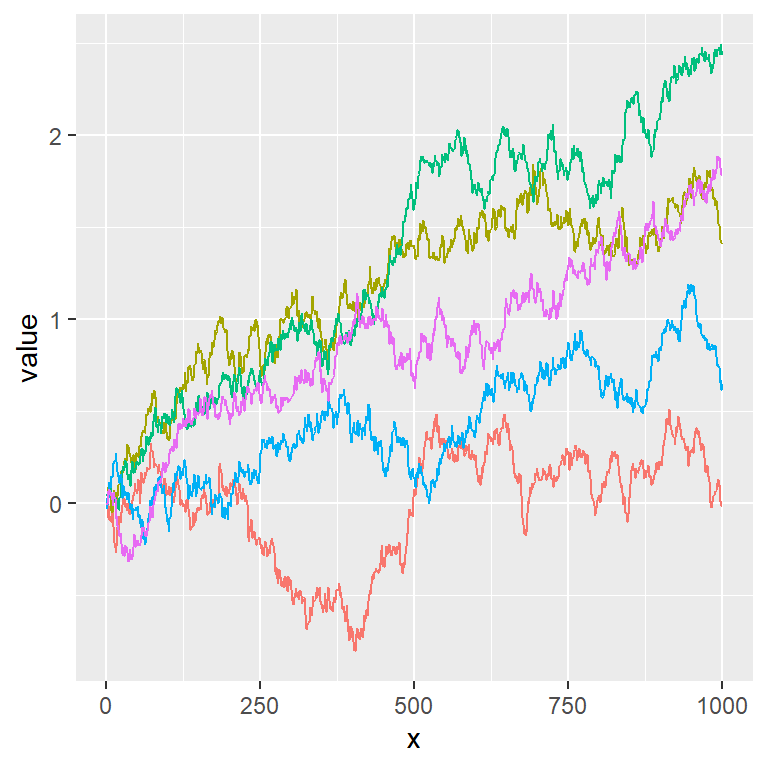Remove the legend

You can also get rid of the legend making use of `legend.position = "none"`.

``````# install.packages("ggplot2")
library(ggplot2)

ggplot(df, aes(x = x, y = value, color = variable)) +
geom_line() +
theme(legend.position = "none")``````